PHYSICS

Mass

WAVE AND PHOTON

WA. Fundamental of Wave WB. Photon WC. Kong Vector
WE. Behaviors of Photon

WD. ENERGY OF PHOTON

1. Kinetic and Potential Energy of Photon 2. Law of Refraction 3. Deform Angle and The Energy of Photon 4. Momentum of Photon
5. Conservation of Momentum 6. Compton Effect

INTRODUCTION

This chapter discusses about the energy of photon in detail. Although photon energy is quantized, under certain condition, the quantized energy of photon can be sub-divided into the kinetic and the potential energy. In this chapter, the detail development and derivation of photon energy is presented.

OBJECTIVES

1)      To establish the kinetic and the potential energy of photon.

2)      To derive the twisting or the deform angle of magnetic and electric components of photon.

3)      To calculate the deform angle and the energy of photon in different traveling medium.

4)   To illustrate the energy transfer by Compton Effect.

## WD.1.0        KINETIC AND POTENTIAL ENERGY OF PHOTON

Photon has linear momentum. Photon travels in vacuum space at the ultimate speed of light. Photon has the quantized energy of hf as given by eq. WD.1.2.

E = hf                                                                                                  … eq. WD.1.1

where   h = Plank’s constant

f = frequency of photon

The energy of photon can be further sub-divided into two portions. There are the kinetic and potential energy of photon. The energy equation of photon is described below,

E = hf = pv + tf                                                                                … eq. WD.1.2

where   p =  momentum of photon

v = traveling speed of photon = the Kong vector

τ = torque, angular force between electric and magnetic component

f = twisting or deform angle of M&E components

When the M&E and Kong vectors are not perpendicular, the photon travels at the lower speed. The kinetic energy of photon is

Kp = pv                                                                                               … eq. WD.1.3

And the potential energy of photon is

PP = τf                                                                                                … eq. WD.1.4

When the M&E and Kong vectors of photon are perpendicular, the photon is traveling at the speed of light. The deform angle of M&E vectors is zero. Therefore, the total energy of photon becomes the kinetic energy, where eq. WD.1.2 becomes

E = Kp = hf = pc                                                                                … eq. WD.1.5

where   c = the speed of photon

When the M&E components of photon are twisted to parallel, the photon is squeezed into a point. The photon is brought to rest and the Kong vector is zero. All the kinetic energy of photon is transformed to the potential energy.

E = Pp = hf = τ(p/2)                                                                           … eq. WD.1.6

When the photon is brought to rest, the full potential energy of photon is also the rest energy of mass.

E = Pp = hf = mc2                                                                              … eq. WD.1.7

where   m = mass of particle

The potential energy of photon is also the Mass Property of the photon. The transformation of photon to particle is discussed in detail in the Chapter ‘Pair Production’ under the main Topic of ‘Mass’.

When photon travels in a medium where the velocity is lower than the speed of light, the photon possesses both the kinetic and the potential energy. Substitute eq. WD.1.5 and eq. WD.1.6 into eq. WD.1.2, we obtain,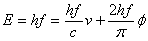… eq. WD.1.8

where   l = wavelength of photon

Simplify eq. WD.1.8, we obtain,… eq. WD.1.9

Rearrange eq. WD.1.9, the deform angle between M&E components of photon is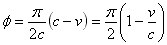… eq. WD.1.10

For photon travels through two different medium with different refraction index, the change of deform angle is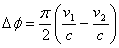… eq. WD.1.11

WD.2.0        LAW OF REFRACTION

From the law of refraction, we know that

n1 sin q1 = n2 sin q2                                                                             … eq. WD.2.1

where   n1 and n2 = the refraction index of medium 1 and 2

q1 and q2 = the incident angle and the refraction angle

If the incoming photon is travel as the speed of light, the refraction index of medium 1 is equal to unity. Hence, the refraction index of medium 2 is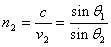… eq. WD.2.2

Substitute eq. WD.2.2 into eq. WD.1.10, we obtain… eq. WD.2.3

Eq. WD.2.3 is the deform angle of photon during refraction.

For two different mediums with different refraction index, from eq. WD.1.11, we obtain,… eq. WD.2.4

WD.3.0        DEFORM ANGLE AND ENERGY OF PHOTON

The higher the refraction index of a medium, the higher the deform angle; the lower the Kong vector; the higher the potential energy and the lower the kinetic energy.

WD.3.1        For Water

Water has the refraction index of n2 = 1.33. When photon enters from the vacuum medium into the water medium, from eq. WD.2.3, the deform angle is… eq. WD.3.1

From eq. WD.2.2, the velocity of photon in the water medium is… eq. WD.3.2

From eq. WD.1.8, consider the yellow sodium photon which has the wavelength of l = 589 nm, the kinetic energy of the photon in the water medium is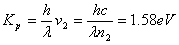… eq. WD.3.3

The potential energy of the yellow sodium photon in the water medium is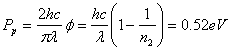… eq. WD.3.4

The total energy of the yellow sodium photon is

E = hf = 2.1eV                                                                                    … eq. WD.3.5

WD.3.2        For Diamond

Diamond has the refraction index of n2 = 2.42. When photon enters from the vacuum medium into the diamond medium, from eq. WD.2.3, the deform angle is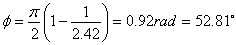… eq. WD.3.6

From eq. WD.2.2, the velocity of photon in the diamond medium is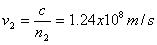… eq. WD.3.7

From eq. WD.1.8, consider the yellow sodium photon which has the wavelength of l = 589 nm, the kinetic energy of the photon in the diamond medium is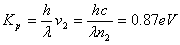… eq. WD.3.8

The potential energy of the yellow sodium photon in the diamond medium is… eq. WD.3.9

The total energy of the yellow sodium photon is

E = hf = 2.1eV                                                                                    … eq. WD.3.10

WD.3.3        Water to Diamond

Water and Diamond has the refraction index of n1 = 1.33 and n2 = 2.42 respectively. When photon enters from water medium to diamond medium, from eq. WD.2.4, the change of deform angle is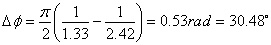… eq. WD.3.11

When photon travels from water medium to diamond medium, the deform angle of photon increases 30.48°, from 22.33° to 52.81°. The kinetic and potential energy are interchanged accordingly without energy loss.

WD.4.0        MOMENTUM OF PHOTON

From eq. WD.1.2, the momentum of the photon is given as follow,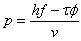… eq. WD.4.1

At free space when the deform angle of M&E components is perpendicular, the photon is travel at the speed of light, eq. WD.4.1 then reduces to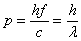… eq. WD.4.2

where   q = 0

c = fl

WD.5.0        CONSERVATION OF MOMENTUM

In any incidents or phenomenon, the principle of conservation momentum of photon shall be complied. Figure WD.5.1 below shows the refraction and reflection of photon when the photon passes through from air medium to glass medium and from glass medium to air medium.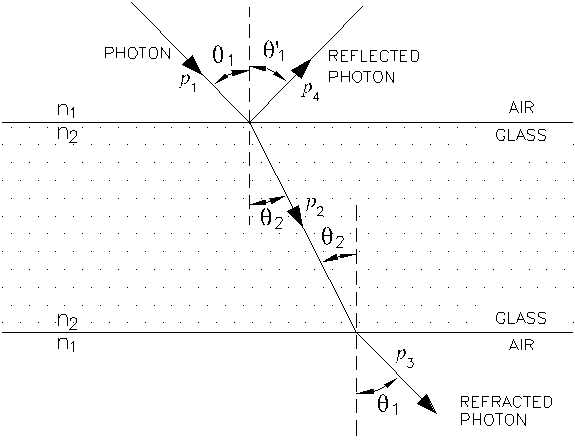Figure WD.5.1

The photon momentum before entering in to glass medium is p1. The reflected portion of photon possesses momentum p4. The refracted portion of photon in glass medium possesses momentum p2 and the final refracted photon to air medium possesses momentum p3.

Figure WD.5.2 below shows another refraction pattern where the second refraction surface is not parallel to the first refraction surface.

WD.5.1        Conservation of Momentum for Refraction

Refraction is the phenomenon where the photon changes direction when it travels from one medium to another medium at different density. Refer to figure WD.5.1, assuming all the incident photons are refracted with reflection, we can write the momentum of photon at air medium before and after refraction as follow,

p1 = p3                                                                                                 … eq. WD.5.1

The momentum of photon before and after shall be the same.

For the conservation of momentum, consequently, the momentum of refracted photon at glass portion shall be also the same, where,

p1 = p2 = p3                                                                                         … eq. WD.5.2

We shall now to prove that the momentum is conserved for refraction in any medium. From eq. WD.4.1, we have the momentum of photon as follow,… eq. WD.5.3

Substitute eq. WD.1.6 into eq. WD.5.3 and rearrange, we obtain,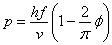… eq. WD.5.4

At any medium with any deform angle, we substitute eq. WD.1.10 into eq. WD.5.4, we obtain,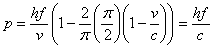… eq. WD.5.5

Eq. WD.5.5 shows that regardless of the travel medium of photon at any deform angle, the momentum of the photon is conserved and only depend on the frequency. Therefore, the eq. WD.5.2 is true and valid. The momentum of refracted photon is conserved in air and glass medium and fully complies to the law of refraction.

WD.5.2        Conservation of Momentum for Reflection

Reflection is the phenomenon where the photon is reflected at the reflective surface. More descriptions on reflection are discussed in the chapter ‘Behaviors of Photon’. Figure WD.5.1 shows the reflection due to the reverse Kong vector. For this type of reflection, the momentum of the photon is conserved and there is no energy loss. To the photon itself, it actually travels in the straight path. From eq. WD.4.2, regardless of the direction, the momentum before and after the reflection is… eq. WD.5.6

The momentum of the system is conserved.

WD.6.0        COMPTON EFFECT

When a photon collides with particle, energy transfers from photon to particle. Photon reduces its energy by reducing the frequency and the wavelength of photon becomes longer.

In Compton Effect, the change of wavelength is named the ‘Compton shift’. The x-ray photon is shot on a target electron. After the collision, the electron is knocked away by the photon and the energy of photon reduces. The collision process adheres to the conservation of energy and momentum. The collision is an elastic collision. The photon energy is reduced and converted to the kinetic energy of knocked electron.

In this case, the photon is particle-like wave-packet. In many cases such as a black object, the photon at visible light range is absorbed by the object. And then, the absorbed energy is emitted at lower energy such as infra-red range. Therefore, a black object looked black, but in actual, black object may emit photons at lower frequency range such as infra-red.

In the cases where the object is not black in color, said red, the energy of photons at visible light range will be absorbed by the electrons of the object, through the same method of collisions. When the excited electrons release energy from higher energy level to lower energy level at the frequency of red light range, the object appears in red color.

DISCUSSIONS AND CONCLUSIONS

The energy of photon can be sub-divided into two portions; they are the kinetic and the potential energy of photon. When the photon is traveling at the speed of light where the M&E vectors of photon are perpendicular, the photon possesses full kinetic energy.

When a photon is brought to rest, the total energy of photon is transformed to the potential energy. This is also named the rest energy of mass.

When photon travels in the transparent medium, the energy of photon can be divided to the kinetic and the potential energy of photon. It is complying to the principle of energy conservation in transparent medium. The same photon travels in the water medium has higher kinetic energy than in the diamond medium and vice versa for the potential energy.

When photon travels in the transparent medium, the momentum of photon in any medium is conserved and complies to the Law of refraction.

The photon losses its energy when collides with particles which able to absorb the photon energy. When photon releases energy, the frequency reduces and the wavelength increases. In visible range, photon at blue color change to yellow or red color after losing energy.

This website is originated on 15-Mar-2007,

updated on 4-Jan-2009.